Learning Library

Time for Times Tables! 10 Printables

Times tables can be tricky. Help fourth graders master multiplication with these math worksheets.

Click on a worksheet in the set below to see more info or download the PDF.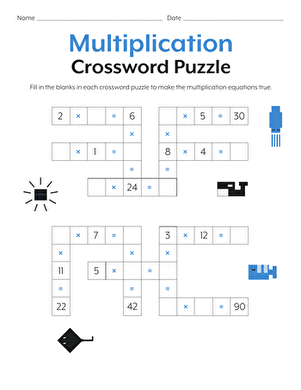Multiplication Crossword

Have some fun reviewing times tables with this multiplication crossword.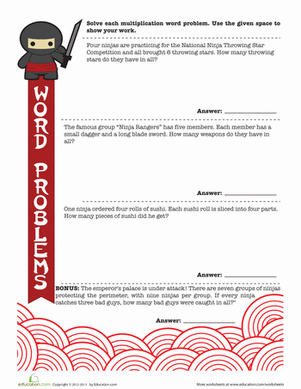Ninja Word Problems

Learn the way of the math ninja with multiplication word problems! Your master mathematician will review times tables as she works through each word problem.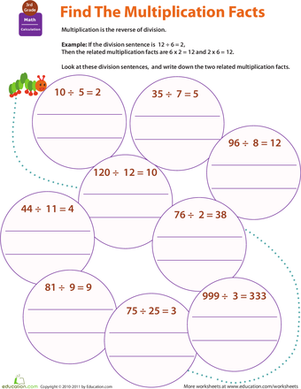Multiplication Facts

Give your fourth grader practice with an important concept: fact families. This one explores the multiplication facts that relate to division facts.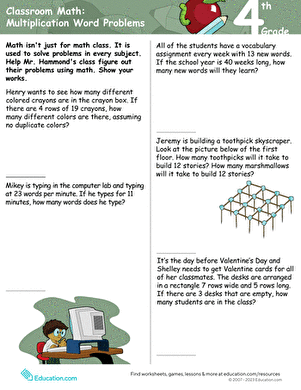Classroom Math: Multiplication Word Problems

Your fourth grader will be challenged to complete five word problems using multiplication with two-digit numbers.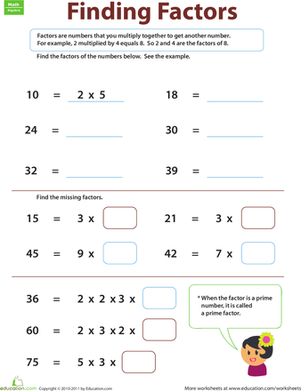Finding Factors

With this worksheet, your child will be learning about factors, and she'll be getting multiplication practice as she goes.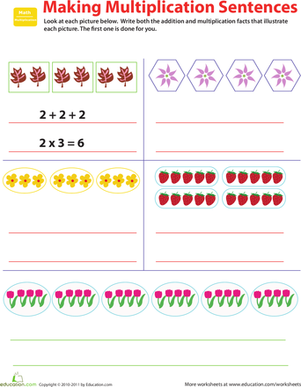Related Facts: Make Multiplication Sentences

Who knew that multiplication and addition were so closely related? Give your fourth grader practice with the concept, with this valuable worksheet.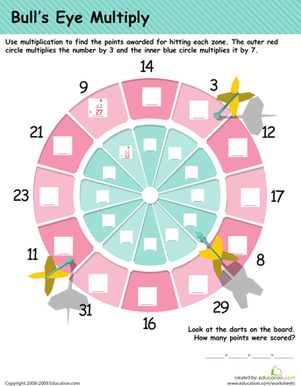Multiplication Practice: Darts!

In this 4th grade math worksheet, your child will practice his multiplication tables as he calculates the points on a dart board.2-Digit Multiplication

Challenge your fourth grader with 2-digit multiplication. Give him a hand practicing multiplying double digits by double digits with this ninja worksheet.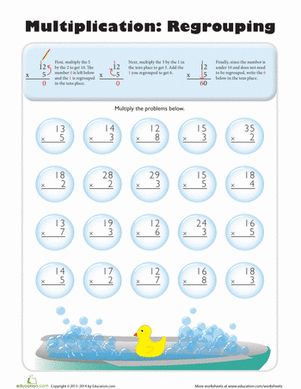Multiplication Regrouping

This worksheet is jam-packed with 2 pages of multiplication regrouping problems to help kids tackle two-digit multiplication.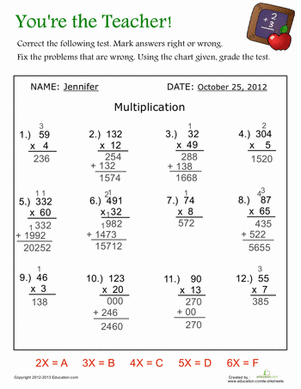You're the Teacher!

No reason to claim the dog ate your homework or an alien abducted your notes -- for this math assignment, you'll be the grader!

Want to download this whole set as a single PDF?

Create new collection

0

New Collection>

0 items

What could we do to improve Education.com?

Please note: Use the Contact Us link at the bottom of our website for account-specific questions or issues.

What would make you love Education.com?